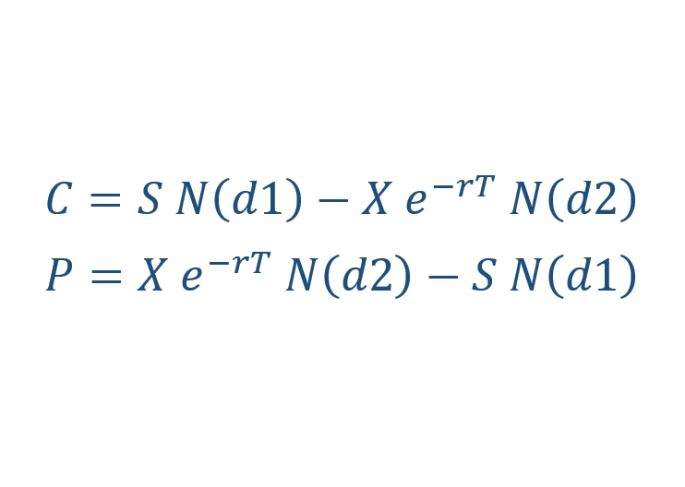## Black-ScholesIntroduction The Black-Scholes formula is the most popular ways to calculate the true price of an option. It is easy to calculate the intrinsic value, but the extrinsic value can be very tricky to calculate. Black Scholes is used for calculating two types of options. Options on stocks Stock Options. Fisher Black, Robert Merton and Myron Scholes originally created the Black Sholes formula in 1973. … Continue reading Black-Scholes »